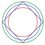# How to make mx+n=0 more complicated?

We all know how to solve the equation $mx+n=0$ ($m,n$ are constants).The answer is of course $-\dfrac nm$.

Then what if one day we forget about how to solve $ax+b=0$,but remember the quadratic formula for $ax^2+bx+c=0$?$x=\frac {-b \pm \sqrt {b^2-4ac}}{2a}$

I have 2 ways:

1.$mx+n=0,x(mx+n)=0$.Solve for $mx^2+nx+0=0$,place $a\rightarrow m,b\rightarrow n,c\rightarrow0$,we get $x=\frac{-n\pm\sqrt{n^2-4m\times0}}{2m}=\frac{-n\pm|n|}{2m}=\frac{-n\pm n}{2m}=0\text{ or }-\frac nm$ That $0$ is of course not a root.So the root is $-\dfrac nm$

2.Solve for $0x^2+mx+n=0$,place $a\rightarrow0,b\rightarrow m,c\rightarrow n$.

Wait,$a$ can't be $0$ ! Try using L'Hopital.

If $m>0$,then $\displaystyle\lim_{a\to 0}\frac{-m-\sqrt{m^2-4na}}{2a}$ doesn't exist. $\lim_{a\to 0}\frac{-m+\sqrt{m^2-4na}}{2a}=\lim_{a\to0}\frac{\dfrac{\partial}{\partial a}(-m+\sqrt{m^2-4na}) }{\dfrac{\partial}{\partial a}( 2a)}=\lim_{a\to0}\dfrac{\dfrac{-4n}{2\sqrt{m^2-4na}}}{2}=\frac{-n}{|m|}=-\frac nm$

If $m<0$,then $\displaystyle\lim_{a\to 0}\frac{-m+\sqrt{m^2-4na}}{2a}$ doesn't exist. $\lim_{a\to 0}\frac{-m-\sqrt{m^2-4na}}{2a}=\lim_{a\to0}\frac{\dfrac{\partial}{\partial a}(-m-\sqrt{m^2-4na}) }{\dfrac{\partial}{\partial a}( 2a)}=\lim_{a\to0}\dfrac{\dfrac{4n}{2\sqrt{m^2-4na}}}{2}=\frac{n}{|m|}=-\frac nm$

Can anyone think of other ways of solving $mx+n=0$?Note by X X
3 years ago

This discussion board is a place to discuss our Daily Challenges and the math and science related to those challenges. Explanations are more than just a solution — they should explain the steps and thinking strategies that you used to obtain the solution. Comments should further the discussion of math and science.

When posting on Brilliant:

• Use the emojis to react to an explanation, whether you're congratulating a job well done , or just really confused .
• Ask specific questions about the challenge or the steps in somebody's explanation. Well-posed questions can add a lot to the discussion, but posting "I don't understand!" doesn't help anyone.
• Try to contribute something new to the discussion, whether it is an extension, generalization or other idea related to the challenge.

MarkdownAppears as
*italics* or _italics_ italics
**bold** or __bold__ bold
- bulleted- list
• bulleted
• list
1. numbered2. list
1. numbered
2. list
Note: you must add a full line of space before and after lists for them to show up correctly
paragraph 1paragraph 2

paragraph 1

paragraph 2

[example link](https://brilliant.org)example link
> This is a quote
This is a quote
    # I indented these lines
# 4 spaces, and now they show
# up as a code block.

print "hello world"
# I indented these lines
# 4 spaces, and now they show
# up as a code block.

print "hello world"
MathAppears as
Remember to wrap math in $$ ... $$ or $ ... $ to ensure proper formatting.
2 \times 3 $2 \times 3$
2^{34} $2^{34}$
a_{i-1} $a_{i-1}$
\frac{2}{3} $\frac{2}{3}$
\sqrt{2} $\sqrt{2}$
\sum_{i=1}^3 $\sum_{i=1}^3$
\sin \theta $\sin \theta$
\boxed{123} $\boxed{123}$

Sort by:

An alternate spin on your approach would be to start with $mx + n = 0$ and square both sides.

$m^2 x^2 + 2 m n x + n^2 = 0 \\ x = \frac{-2 m n \pm \sqrt{4 m^2 n^2 - 4 m^2 n^2}}{2 m^2} = -\frac{n}{m}$

The advantage of this is that we don't have to artificially neglect any roots

- 3 years ago

Good one!I haven't think of this

- 3 years ago

We could multiply both sides by $mx - n$. Then $(mx + n)(mx - n) = 0(mx - n)$ becomes $m^2x^2 - n^2 = 0$, and using the quadratic formula this solves to $x = \frac{-0 \pm \sqrt{0^2 - 4m^2(-n^2)}}{2m^2}$ or $x = \pm \frac{n}{m}$. Then checking for extraneous solutions, we would eliminate $x = \frac{n}{m}$ and keep $x = -\frac{n}{m}$.

- 3 years ago

Thank you.I haven't think of this one.

- 3 years ago

If we can remember how to graph, we could also just graph y = mx + n and find the x-intercept.

- 3 years ago

Ha Ha,that's true!

- 3 years ago